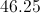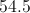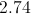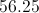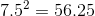# AP Statistics : How to find the standard deviation for a set of data

## Example Questions

### Example Question #1 : How To Find The Standard Deviation For A Set Of Data

A bird watcher observed how many birds came to her bird feeder for four days.  These were the results:

Day 1: 15

Day 2: 12

Day 3: 10

Day 4: 13

Which answer is closest to the standard deviation of the number of birds to visit the bird feeder over the four days?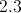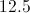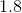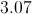Explanation:

Standard deviation is essentially the average distance from the mean of a group of numbers.  There are a number of steps in computing standard deviation, but the steps are not too complicated if you take them one at a time.  First, find the mean of the values.  Second, subtract the mean from the first value and square the result.  Do this for all remaining values.  Third, add these results together.  Fourth, divide this value by the number of values.  Finally, find the square root of the result.

1: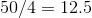2: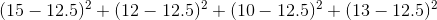3: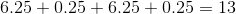4: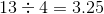5: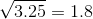### Example Question #2 : How To Find The Standard Deviation For A Set Of Data

Alice recorded the outside temperature at noon each day for one week. These were the results.

Monday: 78

Tuesday: 85

Wednesday: 82

Thursday: 84

Friday: 82

Saturday: 79

Sunday: 80

What is the standard deviation of the temperatures?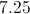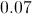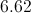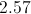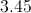Explanation:

There are five steps to finding the standard deviation of a group of values. First, find the mean of the values.  Second, subtract the mean from the first value and square the result.  Do this for all remaining values.  Third, add these results together.  Fourth, divide this value by the number of values minus one.  Finally, find the square root of the result.

### Example Question #1 : Understanding Variance

A bird watcher observed how many birds came to her bird feeder for four days.  These were the results:

Day 1: 15

Day 2: 12

Day 3: 10

Day 4: 13

What is the variance of the number of birds that visited the bird feeder over the four days?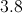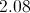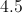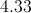Explanation:

Variation measures the average difference between values within a group.  The process is not complicated but there are four steps that can take time.  First, find the mean of the values.  Second, subtract the mean from the first value and square the result.  Do this for all remaining values.  Third, add these results together.  Fourth, divide this value by the number of values in the group minus one (in this case, there are four days).

1:2:3:4: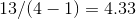Note that to find the standard deviation, we would simply take one additional step of finding the square root of the variance.

### Example Question #2 : Understanding Variance

Alice recorded the outside temperature at noon each day for one week. These were the results.

Monday: 78

Tuesday: 85

Wednesday: 82

Thursday: 84

Friday: 82

Saturday: 79

Sunday: 80

What is the variance of the temperatures?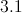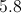Explanation:

There are four steps to finding the variance of values within a group.  First, find the mean of the values.  Second, subtract the mean from the first value and square the result.  Do this for all remaining values.  Third, add these results together.  Fourth, divide the result  by the number of values in the group minus one (in this case, there are seven days, so you must divide by six).

### Example Question #3 : How To Find The Standard Deviation For A Set Of Data

The average height offemales in a class isinches, with a standard deviation ofinches. In the same class, the average height ofboys isinches, with a standard deviation ofinches. What is the mean height of both males and females?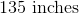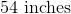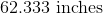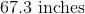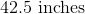Explanation:

To find the mean of the whole population, multiply the female's average by the number of females, and then multiply the male's average by the number of males. Sum up these products and divide by the total number of males AND females: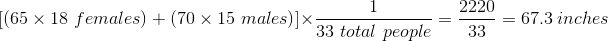### Example Question #3 : Understanding Variance

The standard deviation of a population is 7.5.  What is the variance of the population?